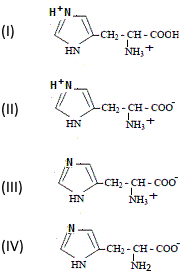<Search:

# Amino acid acid constants

Titration of an amino acid Here is the evolution curve of the $pH$ when titrating the amino acid histidine (weak acid!) with a sodium hydroxide solution:

<Interpretation The →       theory of titration shows that the values of the $pH$ at the half-equivalence points are equal to the $pKa$ of the pairs present. Withwe have: $pKa_{(I)/(II)}\;=\;1.8$ $pKa_{(II)/(III)}\;=\;6.0$ $pKa_{(III)/(IV)}\;=\;9.0$